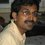# Roots of Identity Matrix

I was reading through some problems based on permutations. Particularly, the following problem interested me.

Let $\mathbb{Z}_n$ denote the set of integers $\{1,2,\ldots,n\}$ for any positive integer $n$ and let $f : \mathbb{Z}_n \rightarrow \mathbb{Z}_n$ be a bijective function defined on $\mathbb{Z}_n$.

Given that $n$ is finite, does integer $k^*$ exist, such that it is always possible to find a $k \le k^*$ for which $f^k(x)=x, \forall x \in \mathbb{Z}_n$?

I suspect that this would turn out to finding the primitive real roots of the identity matrix. That is finding the maximum value of $k$ such that $X^k=I_n$ has a $n \times n$ matrix solution $X$ , but, $X^i \neq I_n$ for any $i < k$

Taking into account that any arbitrary permutations could consist of $m$ cycles of length $i_1,i_2,\cdots,i_m$ with $i_1+i_2+\cdots+i_m=n$. The problem now boils down to finding the maximum value of the LCM of $i_1,i_2,\cdots,i_m$ over all possible values of $m$ i..e,

$k^* = \max_{\substack{m=1\cdots n,\\ i_1+i_2+\cdots+i_m=n}} LCM (i_1,i_2,\cdots,i_m)$ But, am at a loss in actually proceeding much further.Note by Janardhanan Sivaramakrishnan
5 years, 1 month ago

This discussion board is a place to discuss our Daily Challenges and the math and science related to those challenges. Explanations are more than just a solution — they should explain the steps and thinking strategies that you used to obtain the solution. Comments should further the discussion of math and science.

When posting on Brilliant:

• Use the emojis to react to an explanation, whether you're congratulating a job well done , or just really confused .
• Ask specific questions about the challenge or the steps in somebody's explanation. Well-posed questions can add a lot to the discussion, but posting "I don't understand!" doesn't help anyone.
• Try to contribute something new to the discussion, whether it is an extension, generalization or other idea related to the challenge.

MarkdownAppears as
*italics* or _italics_ italics
**bold** or __bold__ bold
- bulleted- list
• bulleted
• list
1. numbered2. list
1. numbered
2. list
Note: you must add a full line of space before and after lists for them to show up correctly
paragraph 1paragraph 2

paragraph 1

paragraph 2

[example link](https://brilliant.org)example link
> This is a quote
This is a quote
    # I indented these lines
# 4 spaces, and now they show
# up as a code block.

print "hello world"
# I indented these lines
# 4 spaces, and now they show
# up as a code block.

print "hello world"
MathAppears as
Remember to wrap math in $$ ... $$ or $ ... $ to ensure proper formatting.
2 \times 3 $2 \times 3$
2^{34} $2^{34}$
a_{i-1} $a_{i-1}$
\frac{2}{3} $\frac{2}{3}$
\sqrt{2} $\sqrt{2}$
\sum_{i=1}^3 $\sum_{i=1}^3$
\sin \theta $\sin \theta$
\boxed{123} $\boxed{123}$